# VLOOKUP With Multiple CriteriaArticle byJeevan A Y## What Is VLOOKUP With Multiple Criteria?

The VLOOKUP function in Excel retrieves the first matching record and stops the search. It allows us to retrieve only one lookup_value from the data table.The VLOOKUP With Multiple Criteria helps us retrieve the required information when the lookup_value contains multiple values.

For instance, look at the following data in Excel.

We are looking to find the sales value for the salesperson “Peter” in the table array A2:C10, which appears 3 times. Here, the VLOOKUP function retrieved the first found “Peter” sales value, i.e., \$6,045. The other 2 two entries are not considered.

Now, we are not sure which “Peter” sales value we require. So, to get an accurate result, we consider the next column after the salesperson column, i.e., the “City” column.

Using the VLOOKUP with multiple criteria, we can specify the city name along with the salesperson’s name.

Now, we are looking for the salesperson “Peter” from the city “Melbourne”. However, this isn’t the right way because we need a lookup column in the combination of salesperson and city in the table array.

###### Key Takeaways
• The VLOOKUP with multiple criteria helps users get the required results for multiple lookup values.
• Using the helper column, we can match multiple criteria columns and find the corresponding value from the table.
• If we do not want helper columns, we can use the alternative methods by using a few Excel functions such as CHOOSE(), MATCH(), IF(), etc.
• The CHOOSE function can select multiple values based on the index number provided in the curly brackets.
• We can match multiple criteria or combinations of column values with the drop-down option in the data validation feature.

### VLOOKUP Multiple Criteria Using Helper Column

As seen in the above example, we do not have a lookup_value column to apply VLOOKUP to search with multiple criteria. In such scenarios, we create a helper column in the data table to assist our multiple criteria for VLOOKUP.

The steps to create a Helper column are,

1. Insert a new column before the first column of the table, and name it “Helper”, i.e., column A.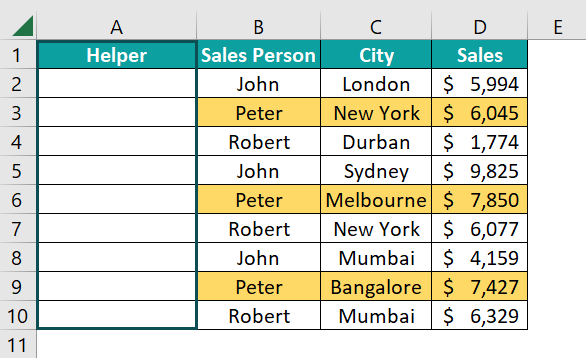2. Now, we must concatenate the “Salesperson” and “City” columns, as shown below.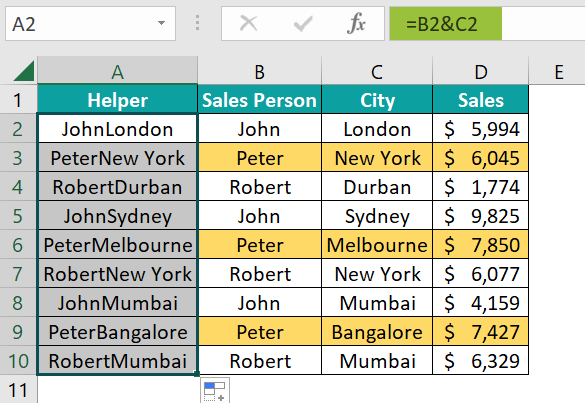First, we select the salesperson column cell, and then we use the ampersand (&) symbol before we select the city column cell. It has created a new column with values combined from the salesperson and city columns. It will be the lookup_value column for the VLOOKUP function.

3. Enter the VLOOKUP function in cell H2.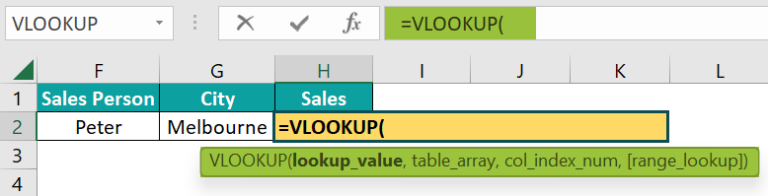4. Now, we must select the lookup_value, similar to the helper column we created. So, choose the salesperson and city column together using the ampersand (&) symbol.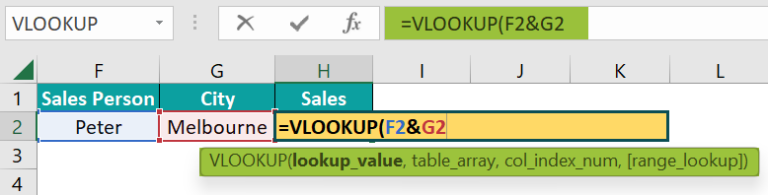5. Choose the table array from A2:D10.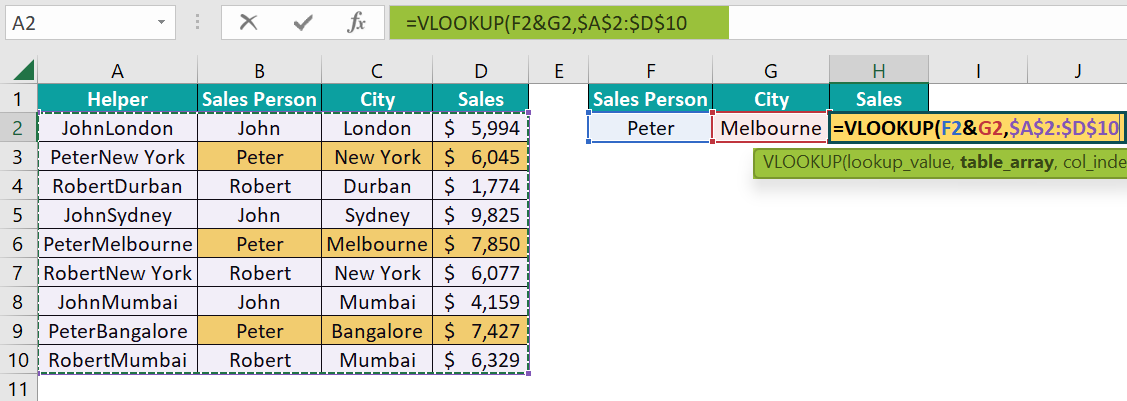6. Enter the column index number as 4 and range lookup as FALSE or 0 to get the exact match.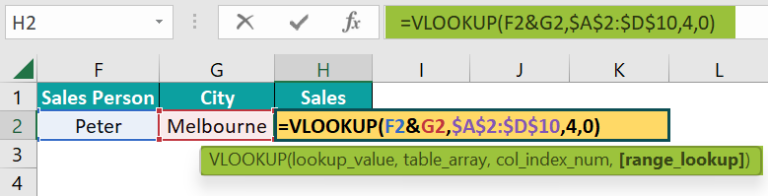7. Close the bracket, and press the “Enter” key to get the result.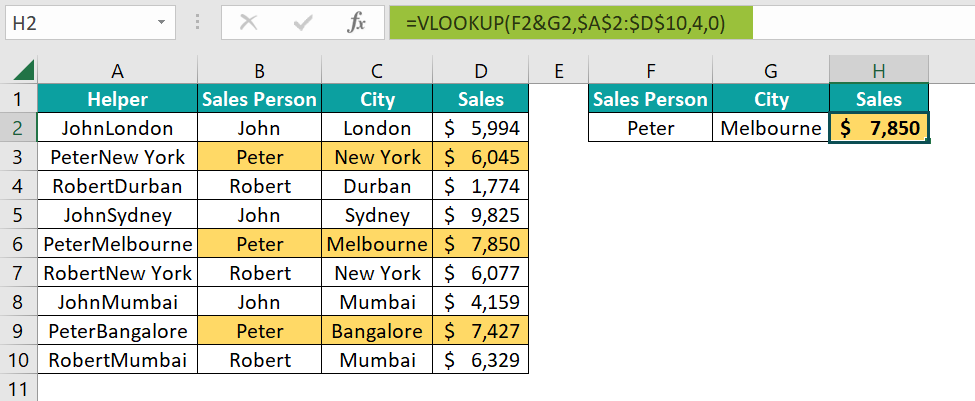Now the VLOOKUP function retrieves the sales value based on multiple criteria. We are seeing the sales value for the salesperson “Peter” and the city “Melbourne”, i.e., \$7,850.

### VLOOKUP Multiple Criteria Using Advanced Formulas & Functions

We can use the VLOOKUP Multiple Criteria using some advanced functions as follows:

1. Using Ampersand to Join Multiple Criteria.
2. CHOOSE function to Join Multiple Criteria in Excel.
3. MATCH function to Include Multiple Criteria in Excel.
4. IF function to Join Multiple Criteria.
5. Multiple Criteria in Single Column in Excel.
6. Use of Drop-Down Lists.

#### Function #1 – Using Ampersand to Join Multiple Criteria

Let us consider another example to create a helper column, i.e., lookup_value column, using the VLOOKUP with multiple criteria.

The following data contains the mobile brand, its version, month, and the number of units sold.

We have another table to retrieve sales units’ values based on the following requirement, i.e., “Mobile Brand”, “Version”, and “Month”.

First, we must create a lookup_value column using the ampersand (&) symbol.

Insert a new column between columns C and D.

Use the ampersand (&) key to combine criteria columns, i.e., “Mobile Brand”, “Version”, and “Month”.

[Note: To get clarity on the combined columns, we have used the pipe (|) symbol between each column.]

The steps to apply the VLOOKUP function, as the lookup_value column is created, are,

• Step 1: Enter the VLOOKUP function in cell K2.
• Step 2: To select the lookup_value, we must choose all the criteria columns using the ampersand (&) symbol. However, before we combine the columns, we must combine the lookup_value cells the same as the lookup_value column created in the table. We use the pipe (|) symbol between each criteria.
• Step 3: Choose the table array from D2:E14.
• Step 4: Enter the column index number as 2, and the matching type as an exact match by entering either FALSE or 0.
• Step 5: Close the bracket, and press the “Enter” key to get the matching result from the multiple criteria by using the ampersand symbol, as shown below.

#### Function #2 – CHOOSE Function to Join Multiple Criteria in Excel

We can use the CHOOSE excel function as an alternative to avoid inserting the helper column.

The syntax of CHOOSE function is,

Here, we must enter the index number, and it will choose the value from the respective position.

Let us take the same data from the earlier example.

For instance, look at the following example.

In the Index argument, we have given 2, and in the values arguments, we have given days of the week.

There are 7 values (days) given, and the CHOOSE function chooses the value from the second position of the sequence of the values, i.e., “Tue”.

Along similar lines, we can combine all the lookup_value columns from the table like the following.

In the index number argument of the CHOOSE function, we have given 1 and 2 in the curly bracket, i.e., when we must select multiple values from multiple positions, we can use the curly bracket and mention the index numbers.

Then, in the value1 argument, we have combined all the criteria columns, i.e., “Mobile Brand”, “Version”, and “Month” columns.

Then in the value2 argument, we have given reference to the “Units” column. Hence, the CHOOSE function is returning the table, as shown below.

We can use the same formula in the table array argument of the VLOOKUP function to avoid creating the helper column.

In the above formula, the CHOOSE function returns the table array as the concatenation of lookup_value columns and unit columns. Then the VLOOKUP function retrieves the value from the table array given by the CHOOSE function.

#### Function #3 – MATCH Function to Include Multiple Criteria in Excel

With the continuation of the previous example, we have added another column to the data table, i.e., Sales Value.

Now we must allow the user to switch between calculations of units and sales value with the same formula by using the MATCH excel function.

In the CHOOSE function, we must select another column, i.e., the Sales Value column.

In the index number, we have given {1,2,3}. The first one is the lookup_value which is the concatenation of columns “Mobile Brand”, “Version”, and “Month”.

Then we have given 2 value columns, i.e., “Units” and “Sales Value”.

So, the CHOOSE function returns the table required for the VLOOKUP’s table array column.

Next, we must dynamically fetch the column index number based on the header of the column.

Here, the MATCH function returns the column index number for the VLOOKUP function based on the column header provided by the user.

If we change the column header to Sales Value, it will return the sales value numbers, as shown below.

#### Function #4 – IF Function to Join Multiple Criteria

The IF excel function is also used to avoid creating the helper column.

Consider the following data to find the sales value based on two criteria, i.e., Salesperson and City.

In our earlier example, we used the helper column to combine the salesperson and city columns to create a unique lookup_value column. However, now we will use the IF condition to create a unique column.

Look at the following image.

In the above formula, the IF condition will test for the city name entered in cell F2, and filter the matching rows from the table range A2:C10.

Since it provides a unique set of values, the VLOOKUP will search for the salesperson in this table, and return the corresponding sales value.

Let us enter this IF condition into the table array argument of the VLOOKUP function.

We get the desired result without adding the helper column, as shown above.

#### Function #5 – Multiple Criteria in Single Column in Excel

We will retrieve the first two matching values for the multiple lookup_values we have, using the VLOOKUP with Multiple Criteria.

For instance, consider the following requirement. We have two salespersons in cells E2 and E3. For these two salespeople, we must find the first two cities.

The following formula will give us the required results.

In the lookup_value argument, we have selected two columns, i.e., E2:E3, and in the table array, we have chosen the range A2:B10. The VLOOKUP function retrieves the first two matching records from the table array, as shown above.

#### Function #6 – Use of Drop-Down Lists

To choose the required lookup_value, we can create drop-down lists to select the lookup_values.

We will use the following data in Excel.

The steps to create a drop-down list for salespeople are,

• Step 1: Choose cell E2 → select the “Data” tab → go to the “Data Tools” group → click the “Data Validation” option drop-down → select the “Data Validation…” option, as shown below.
• Step 2: The “Data Validation” window opens. In the “Settings” tab, go to the “Validation criteria”,
• Choose the “List” option from the drop-down in the “Allow:” field.
• Choose the Sales Person cell range, i.e., A2:A4, in the “Source:” field.
• Step 3: Click “OK” to create a drop-down list of salespeople. Repeat the same for the city cell as well.
• Step 4: Now apply the following VLOOKUP function in cell G2.

Using the drop-down list, we can select the combination of the VLOOKUP, and the IF function will retrieve the value from the table.

### Important Things To Note

• The helper column adds unnecessary data to the table, thus increasing the file size when we work with a large dataset.
• The VLOOKUP retrieves value only for the first matching record when we lookup for one criterion.
• If the correct match isn’t found, the VLOOKUP function will return the #N/A error.
• If we use Excel 2016 and earlier versions, we must execute the formulas as an array excel function using the Ctrl + Shift + Enter keys.
• ALT + A + V + V is the shortcut key to open the data validation window.

1. How to VLOOKUP with multiple criteria using index and match?

The combination of INDEX and MATCH functions is used as an alternative to the VLOOKUP function, and also to match multiple criteria.

For instance, look at the following data in Excel.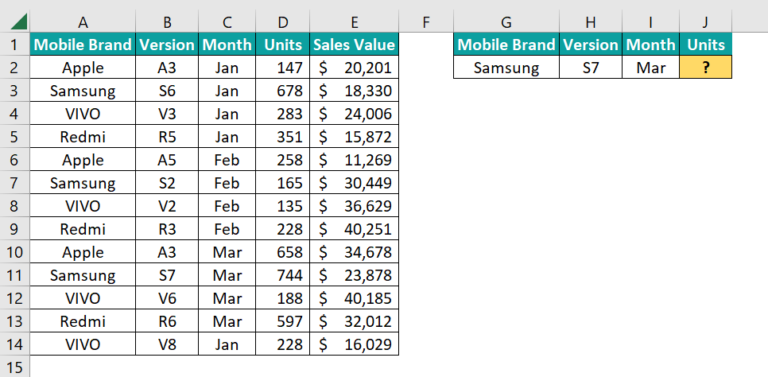Select cell J2, enter the formula
=INDEX(D2:D14,MATCH(1,(G2=A2:A14)*(H2=B2:B14)*(I2=C2:C14),0)), and press “Enter”.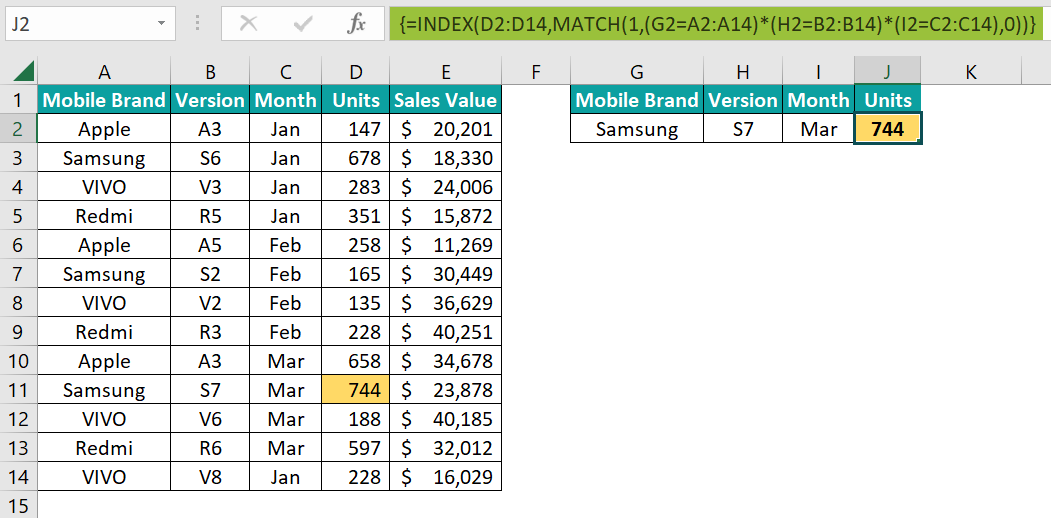It will retrieve the value from the matching combination of all the criteria lookup_values given.

2. How to use VLOOKUP with multiple criteria in different sheets?

To retrieve values from different worksheets, we must create a helper column in the worksheet where the table array is found.

3. What are some limitations of using VLOOKUP with multiple criteria?

Some of the limitations of the VLOOKUP with multiple criteria are:
If we are creating a helper column, then that column should be only to the left of the result column.
The helper column will increase the size of the file in large datasets.

4. Why is VLOOKUP with multiple criteria not working?

If the multiple criterias formula does not return the desired result, we must look at the lookup_value and the concatenation style.